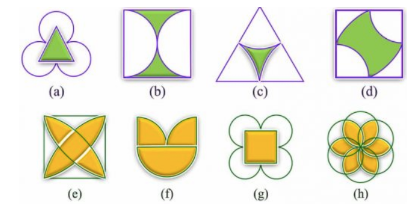# 4.  The following figures have more than one line of symmetry. Such figures are said to have multiple lines of symmetry.Identify multiple lines of symmetry, if any, in each of the following figures:The  lines of symmetry of figures are:

(a)There are 3 lines of symmetry. Thus, it has multiple lines of symmetry.(b) There are 2 lines of symmetry. Thus, it has multiple lines of symmetry.(c)There are 3 lines of symmetry. Thus, it has multiple lines of symmetry.(d)There are 2 lines of symmetry. Thus, it has multiple lines of symmetry.(e)There are 4 lines of symmetry. Thus, it has multiple lines of symmetry.(f)There is 1 line of symmetry.(g)There are 4 lines of symmetry. Thus, it has multiple lines of symmetry.(h)There are 6 lines of symmetry. Thus, it has multiple lines of symmetry.## Related Chapters

### Preparation Products

##### Knockout KCET 2021

An exhaustive E-learning program for the complete preparation of KCET exam..

₹ 4999/- ₹ 2999/-
##### Knockout KCET JEE Main 2021

It is an exhaustive preparation module made exclusively for cracking JEE & KCET.

₹ 27999/- ₹ 16999/-
##### Knockout NEET Sept 2020

An exhaustive E-learning program for the complete preparation of NEET..

₹ 15999/- ₹ 6999/-
##### Rank Booster NEET 2020

This course will help student to be better prepared and study in the right direction for NEET..

₹ 9999/- ₹ 4999/-